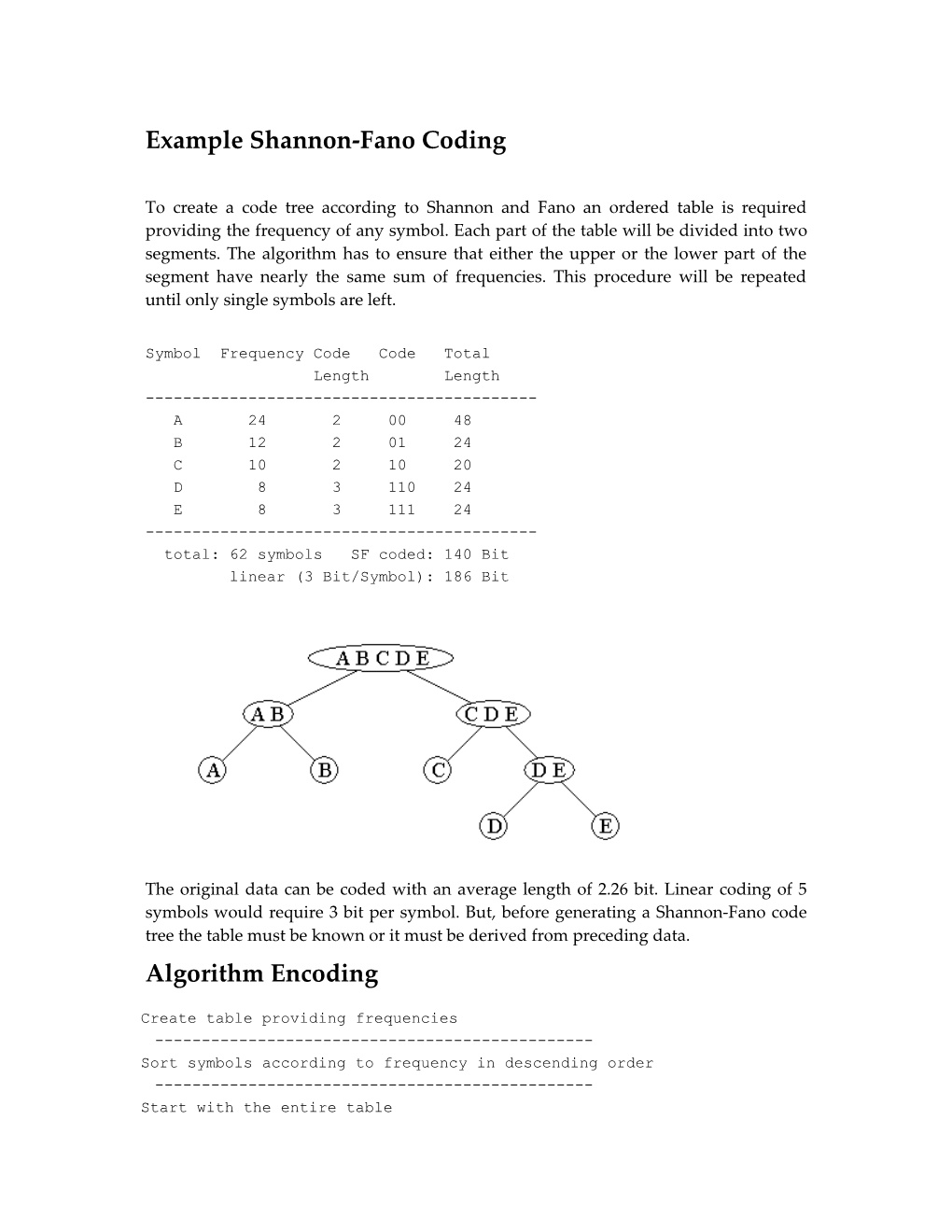# Example Shannon-Fano CodingExample Shannon-Fano Coding

To create a code tree according to Shannon and Fano an ordered table is required providing the frequency of any symbol. Each part of the table will be divided into two segments. The algorithm has to ensure that either the upper or the lower part of the segment have nearly the same sum of frequencies. This procedure will be repeated until only single symbols are left.

Symbol Frequency Code Code Total

Length Length

------

A 24 2 00 48

B 12 2 01 24

C 10 2 10 20

D 8 3 110 24

E 8 3 111 24

------

total: 62 symbols SF coded: 140 Bit

linear (3 Bit/Symbol): 186 BitThe original data can be coded with an average length of 2.26 bit. Linear coding of 5 symbols would require 3 bit per symbol. But, before generating a Shannon-Fano code tree the table must be known or it must be derived from preceding data.

Algorithm Encoding

Create table providing frequencies

------

Sort symbols according to frequencyin descending order

------

------

Division:

------

Seek pointer to the first andlast symbol to the segment

------

Divide the segment into two parts, bothnearly equal in sum of frequencies

------

Add a binary 0 to the code words of theupper part and a 1 to the lower part

------

Search for the next segment containing more than two symbols and repeat division

------

Coding of the origination data according to code words in the table

------

The decoding process follows the general algorithm for interpretation of binary code trees.

Interpretation of Code Trees

A particular code word will be created by running from the root node to the symbol's leaf node. Any left-sided branch adds a 0 to the code word; every right-sided branch a binary 1. The required number of steps or the depth of this part of the code tree is equal to the code length.

The example mentioned above result in the following code representing the three symbols "a", "b" and "c":

0 -- a

10 -- b

11 -- c

Characteristics of Code Trees

Any node in a binary code tree has only one predecessor and two successors. If symbols are assigned only to leaf nodes and interior nodes only construct the tree, it is always sure that no code word could be the prefix of another code word. Such a tree matches the prefix property.

Code trees can be constructed in a way that the probability for the occurrence of the symbols will be represented by the tree structure. A wide-spread procedure is the coding scheme developed by David Huffman.

Binary trees only allow a graduation of 1 bit. More precise solutions will be offered by the arithmetic code.

## Step-by-Step Construction

Freq- 1. Step 2. Step 3. Step

Symbol quency Sum Code Sum Code Sum Code

------

A 24 24 0 24 00

------

B 12 36 0 12 01

------

C 10 26 1 10 10

------

D 8 16 1 16 16 110

------

E 8 8 1 8 8 111

------

### Code trees according to the steps mentioned above:

1.

2.

3.

Shannon-Fano versus Huffman

The point is whether another method would provide a better code efficiency. According to information theory a perfect code should offer an average code length of 2.176bit or 134,882bit in total.

For comparison purposes the former example will be encoded by the Huffman algorithm:

Shannon-Fano Huffman

Sym. Freq. code len. tot. code len. tot.

------

A 24 00 2 48 0 1 24

B 12 01 2 24 100 3 36

C 10 10 2 20 101 3 30

D 8 110 3 24 110 3 24

E 8 111 3 24 111 3 24

------

total 186 140 138

(linear 3 bit code)

The Shannon-Fano code does not offer the best code efficiency for the exemplary data structure. This is not necessarily the case for any frequency distribution. But, the Shannon-Fano coding provides a similar result compared with Huffman coding at the best. It will never exceed Huffman coding. The optimum of 134,882bit will not be matched by both.

Another Example

An Example

Applying the Shannon-Fano algorithm to the file with variable symbols frequencies cited earlier, we get the result below. The first dividing line is placed between the ‘B’ and the ‘C’, assigning a count of 21 to the upper group and 14 to the lower group, which is the closest to half. This means that ‘A’ and ‘B’ will have a binary code starting with 0, while ‘C’, ‘D’, and ‘E’ will have binary codes starting with 1, as shown. Applying the recursive definition of the algorithm, the next division occurs between ‘A’ and ‘B’, putting ‘A’ on a leaf with code 00 and ‘B’ on another leaf with code 01. After performing four divisions, the three most frequent symbols have a 2-bit code while the remaining, rarer symbols have 3-bit codes.

A / 14 / Second Division / 0 / 0
B / 7 / First Division / 0 / 1
C / 5 / Third Division / 1 / 0
D / 5 / Fourth Division / 1 / 1 / 0
E / 4 / 1 / 1 / 1

The binary tree resulting from applying the Shannon-Fano algorithm to the afore-mentioned text file is given below, and differs slightly from the one obtained by applying the Huffman coding to the same exact character set.Note that as with the Huffman tree, since all the characters are situated at the leaves of the tree, the possibility that one code is a prefix of another code is altogether avoided. The table below shows the resultant bit codes for each character:

Symbol / Bit Code
A / 00
B / 01
C / 10
D / 110
E / 111